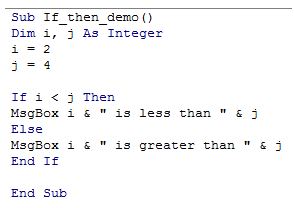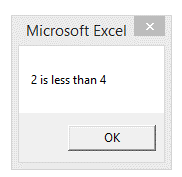## Vba if then else, condition in Excel

No script can be used a achieve a requirement without checking various conditions that must be satisfied.

Excel offers "If Then..Else " for this very purpose.

This statement helps execute a script or part of it based on certain conditions.

Example: Check if a number is graeter than or smaller than the other and execute corresponding code

1. Sub If_then_demo()
2. Dim i, j As Integer
3. i = 2
4. j = 4
5. If i < j Then
6. MsgBox i & " is less than " & j
7. Else
8. MsgBox i & " is greater than " & j
9. End If
10. End Sub

Here is the script from the editorHere is the Result